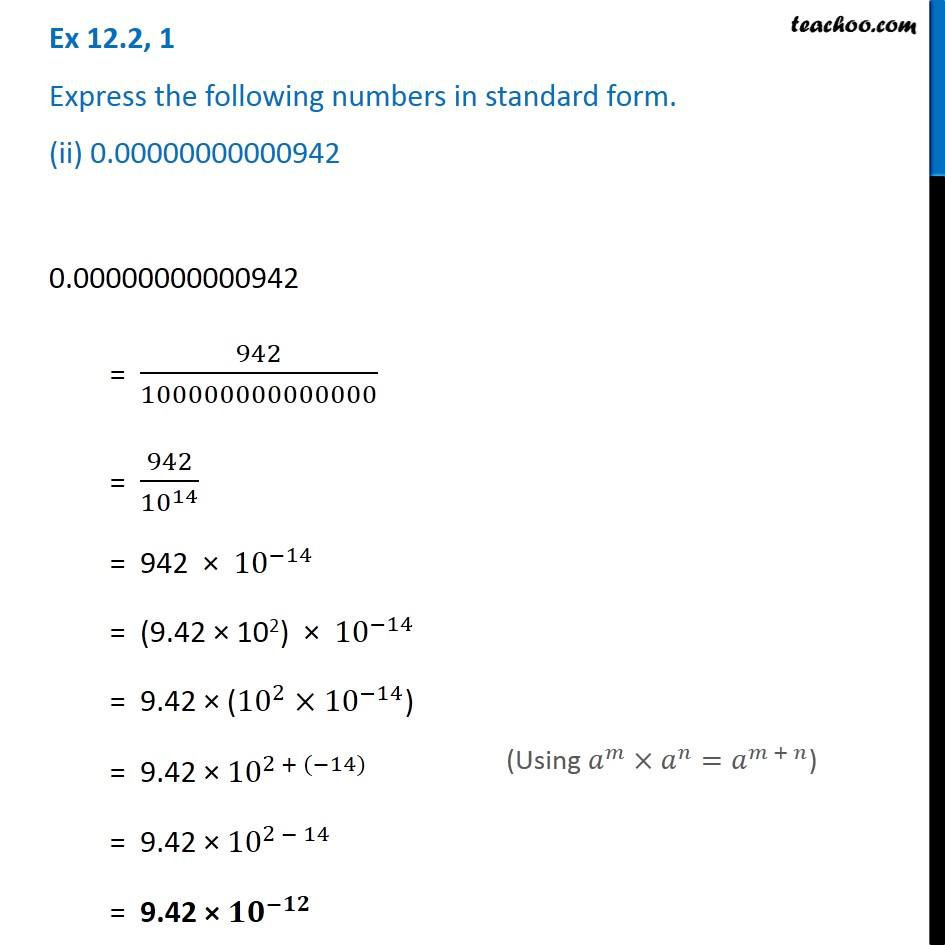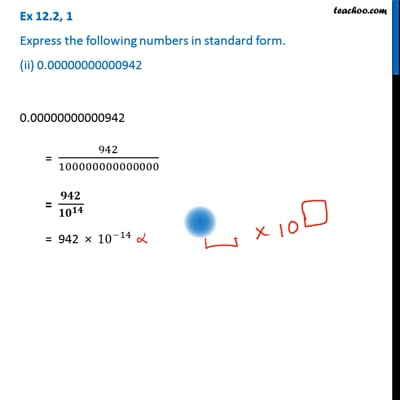Ex 12.2

Chapter 12 Class 8 Exponents and Powers
Serial order wiseThis video is only available for Teachoo black users

Get live Maths 1-on-1 Classs - Class 6 to 12

### Transcript

Ex 12.2, 1 Express the following numbers in standard form. (ii) 0.00000000000942 0.00000000000942 = 942/100000000000000 = 942/〖10〗^14 = 942 × 〖10〗^(−14) = (9.42 × 102) × 〖10〗^(−14) = 9.42 × (〖10〗^2×〖10〗^(−14)) = 9.42 × 〖10〗^(2 + (−14)) = 9.42 × 〖10〗^(2 − 14) = 9.42 × 〖𝟏𝟎〗^(−𝟏𝟐) (Using 𝑎^𝑚×𝑎^𝑛=𝑎^(𝑚 + 𝑛))